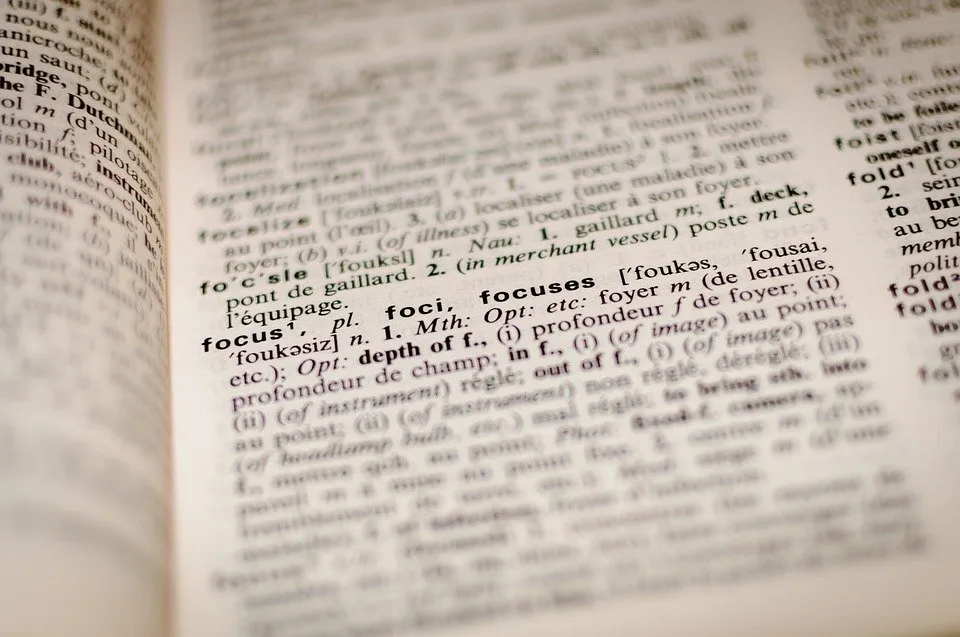# Different Kind Of Wrong Answers In Math

Hi there. In this math & education post, I cover the topic of different kind of wrong answers in math. It is well known that math is a difficult subject. When it comes to math practice, homework, tests and assignments there are actually many ways you can get a math problem wrong. I provide an overview on the different ways one can get wrong answers in mathematics. The contents of this post is based from memory and my experiences as a student and as someone currently in educational services.Pixabay Image Source

## Topics

• Some Answers Are Close to Correct
• Wrong Formulas
• Typos
• Calculation Errors
• Plus Vs Minus Signs
• Not Knowing Definitions
• Not Following Instructions
• Harsh Marking

## Some Answers Are Close to Correct

Mathematics is one of those subjects where you are either right or wrong. When it comes to math learning, there are cases when some of the math answers are close to the correct answer. There are also cases when some of the answers from students are not even close to the correct answers from answer keys or from the teacher's point of view. The later sections in this post looks at different cases of wrong answers.

Note that there are some math fields which involve less numbers and more of logic and writing. With math proofs for example, an answer can be considered correct depending on the math professor's opinion on the student's math proof, reasoning and writing.Pixabay Image Source

Having blank answers in math on a test or assignment is not good. Here are some possible reasons on someone having blank answers.

• Not knowing the material
• Not knowing how to answer the question
• Ran out of time to do the question
• An actual difficult question
• Possible language barriers

## Wrong Formulas

One mistake that happens sometimes is using the wrong math formula to solve a problem. As an example, the formula for the volume of a cylinder and the volume of a cone are similar but there is the 3 on the denominator for the volume of a cone.Rendered with Quicklatex.com

Mistakes involving the misuse of a formula would not give full marks. Partial marks for a question could be given. It depends on the marking philosophy of the teacher or school.

Some tests allow for formulae sheets or cheat sheets. This is nice as students don't have to focus on memorization and they can focus on understanding. Knowing when to use certain formulas is still important.Pixabay Image Source

## Typos

One of the worst and annoying ways to lose marks is through typos. Writing a 31 instead of the correct answer of 37 is one example. Messy writing does not help those who are marking tests and assignments. It makes it hard for the markers to give marks if answers are hard to read and understand.

Sometimes typos and messy writing occurs when you are rushed. Rushing occurs for those who are running out of time. If possible maybe practice faster writing that is neat enough to read.

## Plus Vs Minus Signs

This case of plus vs minus signs typically happen with younger students. The mistake here is where students add numbers, quantities or fractions when they should subtract and vice versa.

Having `7 + 4 = 11` is correct but `7 - 4 = 11` is not correct. The minus sign is ignored and was treated as a plus sign.Pixabay Image Source

## Calculation Errors

I do think that math calculation errors are common with many math students. These errors can include:

• Plus vs Minus signs
• Exponents vs Multiplication
• Missing Negative Sign
• Incorrect Usage of Algebra
• Missing Symbols
• Incorrect Calculus Derivative/Integral

A good portion of these errors can be from a lack of strong algebra skills. Some of these mistakes could be typos or from a lack of focus. What makes math hard at more higher levels is the level of consistency and precision with algebra needed to do well. A lot of it comes from hard work, strong focus and some grit. Getting things wrong is part of the practice.

## Not Knowing Definitions/Concepts

Not knowing math definitions and concepts can lead to errors in answers. This is a result from not enough studying or simply confusion. Some definitions and concepts are much harder to understand than others. For those who are familiar with pure math with proofs and abstract concepts you would know what I mean.Pixabay Image Source

## Harsh Marking

There are cases when wrong answers are heavily punished. Partial marks may not be given as effort is not rewarded. Tests could be under the multiple choice format where the answer is either or wrong. It does not matter how much scrap work or studying you have done in multiple choice tests. Assignments and problem solving questions could also be marked harshly. It could be either in the all or nothing marking format. You could have a simple typo in the answer as in `10pi` instead of the correct answer of `100pi` and not receive full marks.

## Not Following Instructions

As a general thing it is important to follow instructions. This is for tests, assignments, building IKEA furniture, taking medicine, using a food recipe and so on. In the mathematics setting it can be costly to not follow instructions. One of the worst feelings is doing all the hard work with calculations & writing and then figuring out that you missed a key instruction or detail in the question.

It is a good idea to reread questions. There are some questions on tests and assignments that seem very confusing at the first read. Rereading the questions helps digest the questions into the brain. Do consider time management as well. There is only so much time on a test or on an assignment.Pixabay Image Source